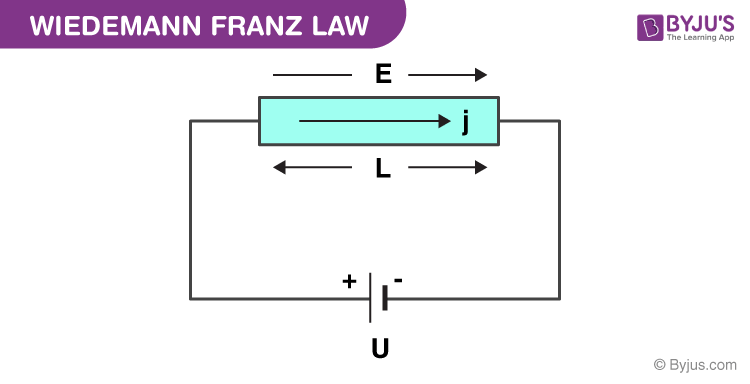# Wiedemann-Franz Law

## What Is Wiedemann-Franz Law?

According to the Wiedemann-Franz law

The ratio of the thermal conductivity of the material and the electrical conductivity of the material is directly relative to the temperature.

The Wiedemann-Franz law is one of the fundamental laws in physics. This law was proposed in the year 1835 and is named after German physicists, Gustav Wiedemann and Rudolph Franz. Gustav Wiedemann discovered that thermal conductivity (κ) and the electrical conductivity (σ) are approximately have same value at the same temperature for different metals.

In this law:

Thermal Conductivity (κ) is the degree of the capacity of a material to conduct heat.

Electrical Conductivity (σ) is the degree of the capacity of a material to conduct electricity 1/ρ.Wiedemann-Franz Law Model

The law states that the ratio of the electronic contribution of the thermal conductivity (κ) to the electrical conductivity (σ) of metal is somewhat equivalent to the temperature (T).

$$\begin{array}{l}\frac{\kappa }{\sigma } = LT\end{array}$$

Here, the L is the proportionality constant, and it is called the Lorenz number. The L is equal to:

$$\begin{array}{l}L = \frac{\kappa }{\sigma T} = \frac{\pi ^{2}}{3}\left ( \frac{\kappa _{B}}{e} \right )^{2} = 2.44 \times 10^{-8} \ W\Omega K^{-2}\end{array}$$

The relationship between the thermal and electrical conductivity is based on the fact that heat and electrical movement involves freely moving electrons in the metal.

The Wiedemann-Franz law is based upon the fact that the heat and electrical transport both involve the free electrons in the metal. It also shows that with increase in particle velocity the electrical conductivity decreases and the thermal conductivity increases with the average particle velocity.

## Wiedemann Franz Law Limitations

1. The value of Lorenz number L will not remain the same for all materials.
1. The law doesn’t hold true for intermediate temperature.
1. The temperature decreases as both (κ) and (σ) increases in the pure metals.